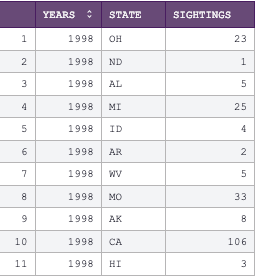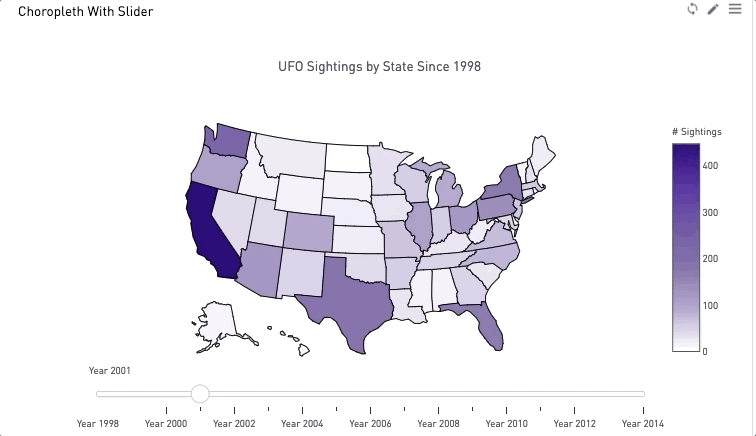# Plotly Choropleth With Slider (Map Charts Over Time)

Plotly is great for its interactivity on visualizations! With choropleth charts we can add a slider to the bottom of the chart to see how data changes over time on a map. The end user can drag the slider across the bottom to see the colors on the heat map change year to year.

Sample of data format:```import numpy as np
import pandas as pd
import plotly.graph_objs as go
import plotly.plotly as py

# min year in your dataset
year = 1998

scl = [[0.0, '#ffffff'],[0.2, '#b4a8ce'],[0.4, '#8573a9'],
[0.6, '#7159a3'],[0.8, '#5732a1'],[1.0, '#2c0579']] # purples

data_slider = []
for year in df['years'].unique():
df_segmented =  df[(df['years']== year)]

for col in df_segmented.columns:
df_segmented[col] = df_segmented[col].astype(str)

data_each_yr = dict(
type='choropleth',
locations = df_segmented['state'],
z=df_segmented['sightings'].astype(float),
locationmode='USA-states',
colorscale = scl,
colorbar= {'title':'# Sightings'})

data_slider.append(data_each_yr)

steps = []
for i in range(len(data_slider)):
step = dict(method='restyle',
args=['visible', [False] * len(data_slider)],
label='Year {}'.format(i + 1998))
step['args'][i] = True
steps.append(step)

sliders = [dict(active=0, pad={"t": 1}, steps=steps)]

layout = dict(title ='UFO Sightings by State Since 1998', geo=dict(scope='usa',
projection={'type': 'albers usa'}),
sliders=sliders)

fig = dict(data=data_slider, layout=layout)
periscope.plotly(fig)```